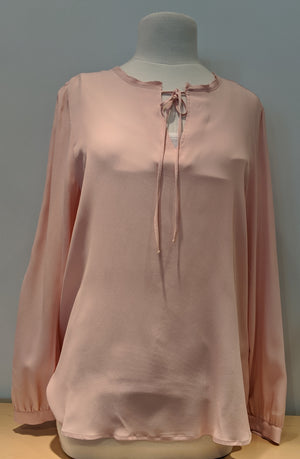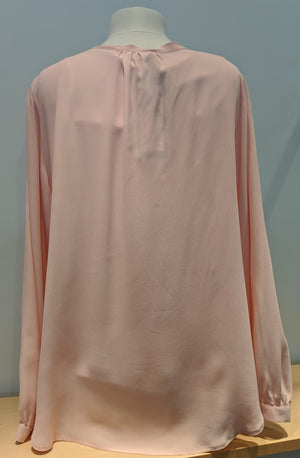Riani

# Blouse

Regular price \$345 now \$172.50 Unit price  per

Shipping calculated at checkout.
• 62% Acetate 38% silk
• Machine wash cold. Hang to dry
• Dry clean
• Simple & elegant silhouette
• Can be worn for multiple occasions
• Tie neck
• Fits true to size

Size Guide

• 34 = 4 = XS
• 36 = 6 = S
• 38 = 8 = M
• 40 = 10 = M
• 42 = 12 = L
• 44 = 14 = L
• 46 = 16 = XL